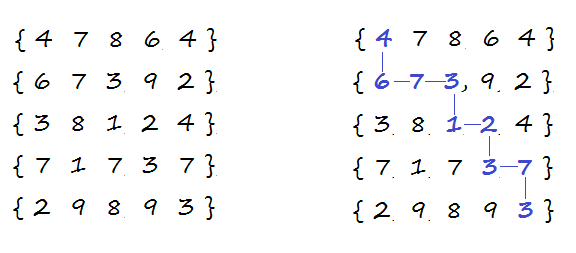# Find the minimum cost to reach last cell of the matrix from its first cell

Given a M x N matrix where each cell has a cost associated with it, find the minimum cost to reach last cell (M-1, N-1) of the matrix from its first cell (0, 0). We can only move one unit right or one unit down from any cell. i.e. from cell (i, j), we can move to (i, j+1) or (i+1, j).

For example,The highlighted path shows the minimum cost path having cost of 36.

The idea is to use recursion. The problem has an optimal substructure. That means the problem can be broken down into smaller, simple “subproblems”, which can further be divided into yet simpler, smaller subproblems until the solution becomes trivial. The problem can be recursively defined as –

Cost to reach cell (m, n) = cost[m][n] + min (cost to reach cell (m, n-1),
cost to reach cell (m, n-1))

Below is C++ and Java implementation of the idea:

## C++

Output:

The minimum cost is 36

## Java

Output:

The minimum cost is 36

The time complexity of above solution is exponential as we’re doing a lot of redundant work. For example, consider the recursion tree for a 5 x 5 matrix.As we can see, the same sub-problems (highlighted in same color) are getting computed again and again. As the recursion grows deeper, more and more of this type of unnecessary repetition occurs. We know that problems having optimal substructure and overlapping subproblems can be solved by dynamic programming, in which subproblem solutions are memoized rather than computed again and again. Now each time we compute the minimum cost of reaching any cell (i, j), we save it. If we are ever asked to compute it again, we simply give the saved answer, and do not recompute it.

The following bottom-up approach computes, for each 0 <= i < M and 0 <= j < N, the minimum costs of reaching cell (i, j) from cell (0, 0), using the costs of smaller values i and j already computed. It has the same asymptotic run-time as Memoization but no recursion overhead.

Below is C++ and Java implementation of the idea:

## C++

Output:

The minimum cost is 36

## Java

Output:

The minimum cost is 36

The time complexity of above solution is O(M x N) and auxiliary space used by the program is O(M x N).

Exercise: Extend the solution to consider diagonal moves as well.(1 votes, average: 5.00 out of 5)Loading...